### Percent Change in MS Excel

You can use the percent change formula in Excel. Percent change can be used to calculate the Monthly , Yearly & Total Change.
Let's learn with example
Here we will calculate the Yearly Percentage change in sales of company A
>> Select cell C5 and enter the formula =(B5-B4)/B4>> Press enter , you will get the value as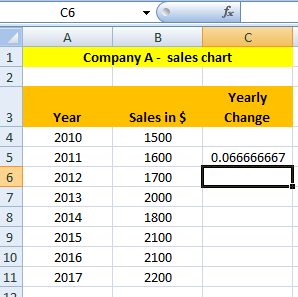>> Select cell C5. On the Home tab, Apply Percentage Format. Or you can press (Ctrl+Shift+%)
(Ctrl+Shift+%) is shortcut to apply percentage format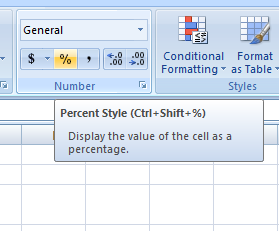Result will be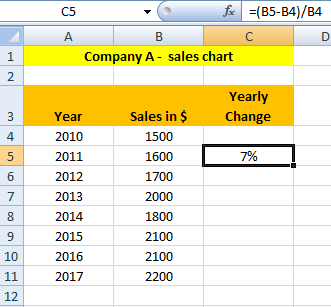>> Now, Select cell C5, click on the lower right corner and drag it down to cell C11.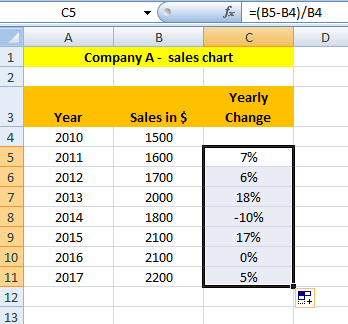Like this way, you can calculate the percentage change .
Let's look at a new example.
Here we will calculate the percent change of marks of students or you can say we will calculate percent change in two values.
>> Select cell D2 and enter the formula =(C2-B2)/B2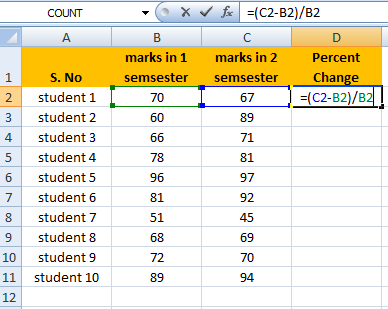>> Press enter , you will get the value as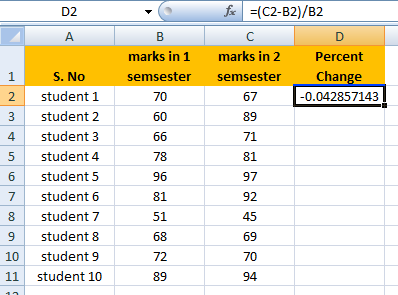>> Select cell D2 On the Home tab, Apply Percentage Format.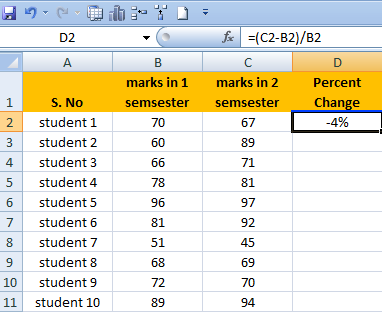>> Now, Select cell D2, click on the lower right corner and drag it down to cell D11.
And the Result will be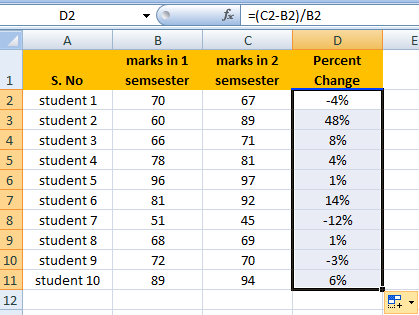Likewise you can calculate any Percent Change you want.